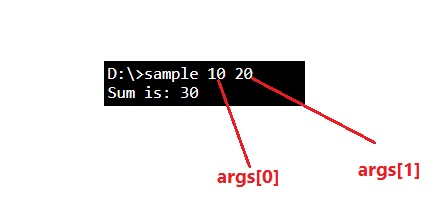# C# - Command-line arguments

In this tutorial, we will learn about the Command-line arguments in C# with the help of examples, how does it work with examples? By IncludeHelp Last updated : April 06, 2023

## What are command-line arguments in C#?

In C#, the command-line arguments are used to pass the values from the user or programmer to the program (or, Main() method). This is the way for message/value passing between the user to the Main() method that can be used within the method.## Passing arguments (values) to Main() method

The arguments are passed during executing the program through the command line. Consider the below statements (file name is "hello.cs".

```Compile: csc hello.cs
Execute: hello.exe Raghav 21
```

Here, "Raghav" and 21 are the values to be passed.

## Command-line arguments Example in C#

```using System;

class Sample {
static void Main(string[] args) {
int SUM = 0;
int X = 0;
int Y = 0;

X = Convert.ToInt32(args);
Y = Convert.ToInt32(args);

SUM = X + Y;

Console.WriteLine("Sum is: " + SUM);
}
}
```

### Output

```D:\>sample 10 20
Sum is: 30
```

### Explanation

In this program, args of main function accepts argument values from console when we execute the program.

Here, we are passing 10 and 20 as command line arguments which can be accessed through args. Where 10 will be assigned into args and 20 will be assigned into args.